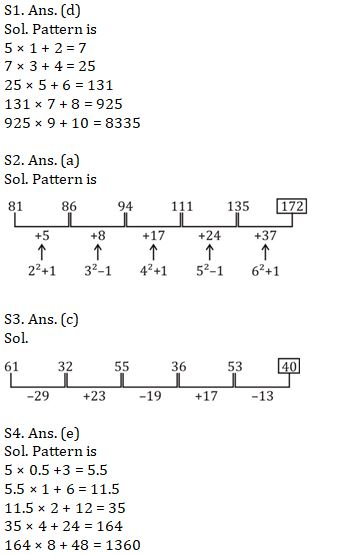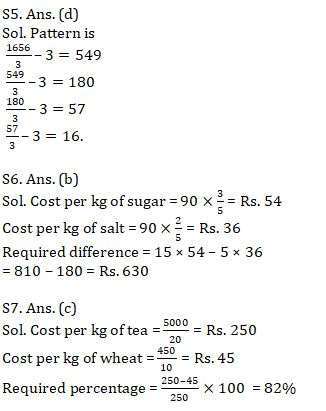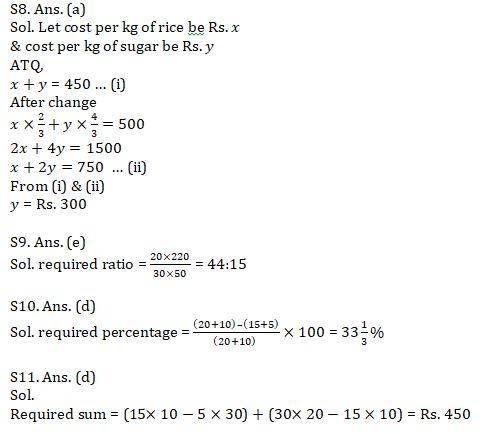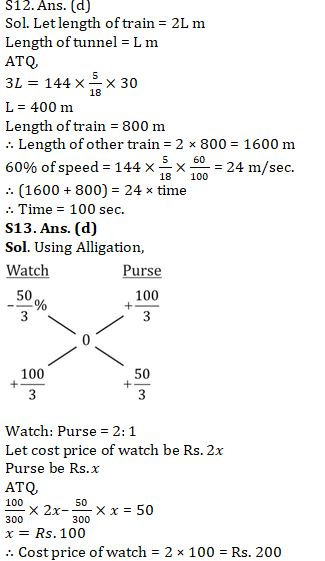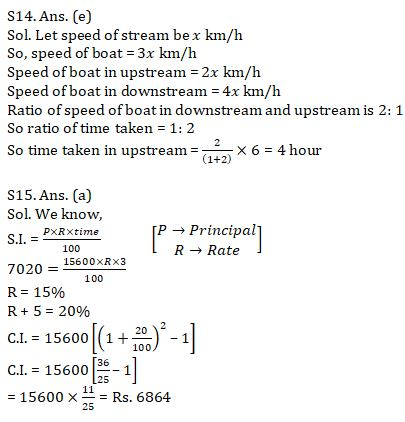Latest Banking jobs   »   Quantitative Aptitude Quiz For IBPS RRB...

# Quantitative Aptitude Quiz For IBPS RRB PO, Clerk Prelims 2021- 13th August

Directions (1-5): What will come in the place of the question mark (?) in the following number series?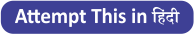Q1. 5, 7, 25, 131, ? , 8335
(a) 845
(b) 940
(c) 965
(d) 925
(e) 825

Q2. 81, 86, 94, 111, 135, ?
(a) 172
(b) 176
(c) 192
(d) 182
(e) 186

Q3. 61, 32, 55, 36, 53, ?
(a) 50
(b) 42
(c) 40
(d) 65
(e) 48

Q4. 5, ? , 11.5, 35, 164, 1360
(a) 7.5
(b) 11
(c) 8.5
(d) 9
(e) 5.5

Q5. 1656, 549, 180, ? , 16
(a) 63
(b) 73
(c) 85
(d) 57
(e) 67

Directions (6-11): Line graph shows the quantity of 5 different products purchased by a person.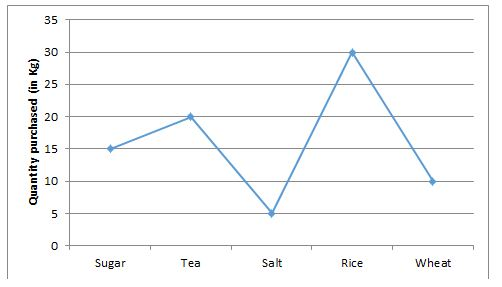Q6. If sum of per kg cost of sugar and that of salt is Rs.90 and the ratio between per kg cost of sugar and that of salt is 3 : 2. Then, find the difference of total cost of sugar and total cost of salt ?
(a) Rs. 530
(b) Rs. 630
(c) Rs. 670
(d) Rs. 750
(e) Rs. 720

Q7. If total cost of Tea is Rs. 5000 and that of wheat is Rs. 450. Then cost per kg of wheat is what percent more or less than cost per kg of Tea ?
(a) 72%
(b) 86%
(c) 82%
(d) 78%
(e) 92%

Q8. One kg of rice and one kg of sugar is purchased in Rs 450. If cost per kg of rice decreases by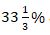& cost per kg of Sugar increases bythen total cost per kg of rice and sugar is Rs. 500. Then find cost per kg of sugar ?
(a) Rs. 300
(b) Rs. 350
(c) Rs. 200
(d) Rs. 250
(e) Rs. 450

Q9. If cost per kg of Tea & per kg of Rice is Rs. 220 & Rs. 50 respectively then find the ratio of total cost of tea to total cost of rice ?
(a) 53 : 15
(b) 44 : 17
(c) 41 : 17
(d) 47 : 15
(e) 44 : 15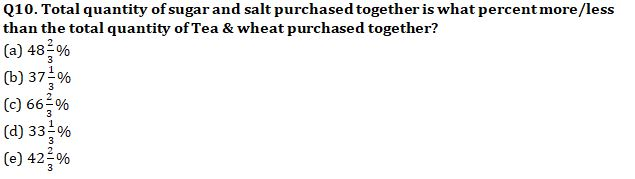Q11. If cost per kg of sugar, salt & rice is Rs. 10, Rs. 30 & Rs. 20 respectively then find the sum of difference of total cost of sugar and that of salt and difference of total cost of sugar and that of rice?
(a) Rs. 500
(b) Rs. 475
(c) Rs. 400
(d) Rs. 450
(e) Rs. 435

Q12. A train crosses a tunnel which is half of its length with a speed of 144 km/hr. in ½ min, then find the time in which it will cross another train which is double of its length and standing on platform in opposite direction with 60% of its initial speed ?
(a) 120 sec.
(b) 90 sec.
(c) 150 sec.
(d) 100 sec.
(e) 180 sec.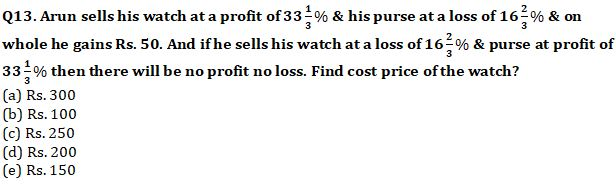Q14. A boat can cover an equal distance in upstream and in downstream in 6 hours. If speed of boat in still water is 200% more than the speed of stream then find the time taken to cover the same distance in upstream.
(a) 5 hours
(b) 3 hours
(c) 4.5 hours
(d) 3.5 hours
(e) 4 hours

Q15. Prabhat invested Rs. 15600 on SI at rate of R% p.a. for 3 years & the interest obtained is Rs. 7020. If he invested the same amount at rate of (R+5)% p.a. for two years on CI then find the interest obtained by Prabhat?
(a) Rs. 6864
(b) Rs. 6250
(c) Rs. 6748
(d) Rs. 6468
(e) Rs. 6648

Practice More Questions of Quantitative Aptitude for Competitive Exams:

###### Target IBPS RRB PO and Clerk Prelims 2021

Solutions